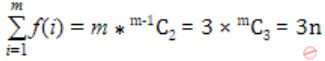## Sets and Functions

 Question 1

Let S = {1,2,3,....,m}, m > 3. Let x1, x2,....xn be the subsets of S each of size 3. Define a function f from S to the set of natural numbers as, f(i) is the number of sets of Xj that contain the element i. That is f(i) = |{j|i ∈ Xj|}|.

Thenis

 A 3m B 3n C 2m+1 D 2n+1
Engineering-Mathematics       Sets And Functions       GATE 2006
Question 1 Explanation:There is 1 question to complete.

Register Now